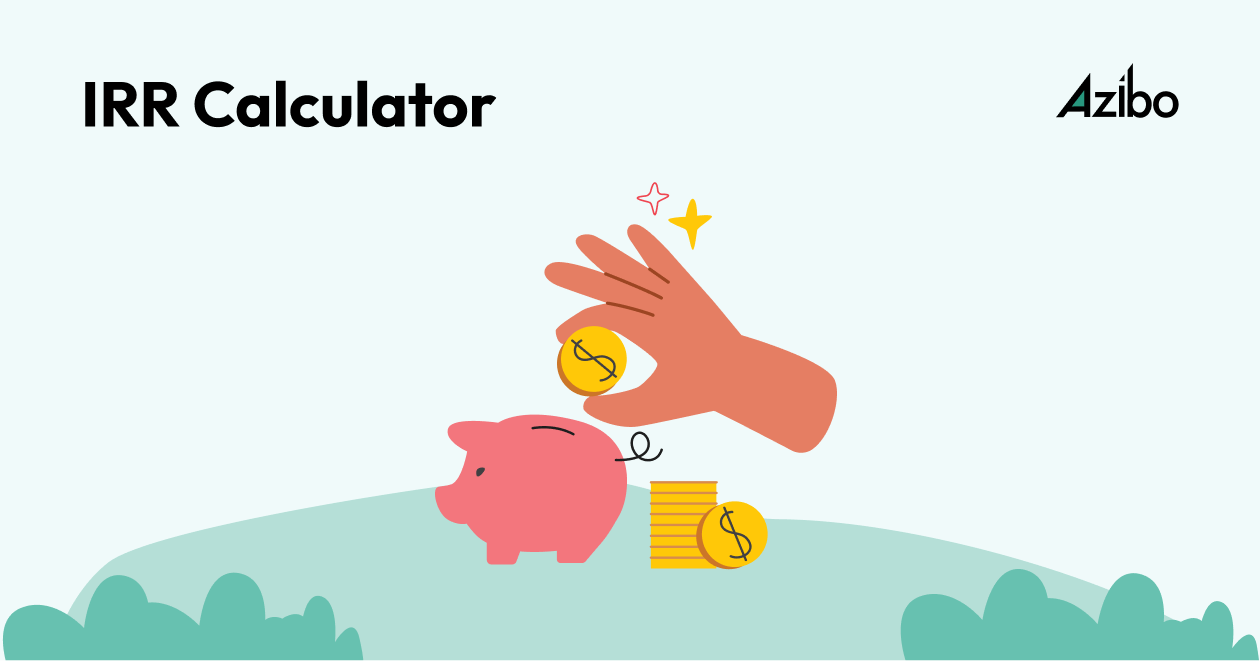March 26, 2023

# Internal Rate of Return Calculator: How to Calculate IRR & What it Means

Determine your IRR (internal rate of return) for a single cash flow## What is IRR (Internal Rate of Return)?

Internal Rate of Return (IRR) is a financial metric used to evaluate the profitability of an investment by estimating the annualized return it is expected to generate over a given period. It is a discount rate that makes the Net Present Value (NPV) of all cash flows from a particular investment equal to zero.

In other words, IRR is the rate at which an investment breaks even in terms of NPV. It is widely used in capital budgeting, financial analysis, and investment appraisal to compare the desirability of different investment opportunities.

## How is IRR Calculated?

Calculating the Internal Rate of Return involves finding the discount rate that results in an NPV of zero for a series of cash flows. The cash flows typically include an initial investment followed by a series of positive cash inflows generated by the investment. The IRR calculation is iterative and usually requires specialized financial software, spreadsheet functions, or custom algorithms.

The IRR formula is:

0 = NPV = ∑ [ CF_t / (1 + IRR)^t ]

Where:

• NPV is the Net Present Value
• CF_t is the cash flow at time t
• IRR is the Internal Rate of Return
• t is the time period

To calculate IRR, you can use several methods such as the trial-and-error method, interpolation, or more advanced numerical methods like the Newton-Raphson method. Some spreadsheet software, like Microsoft Excel, provides built-in functions (e.g., IRR()) that can help calculate IRR easily.

## Why is IRR Important for Real Estate Investors?

When it comes to quickly analyzing and comparing potential investment properties, investors have a few arrows in the quiver such as cash on cash return, cap rate, and gross rent multiplier. The internal rate of return is another such metric that can be used in a similar way - and just like the aforementioned methods, IRR should be just one of the several considerations you should be thinking about when weighing potential investments against each other.

IRR is a great tool for the following use cases:

• Risk Analysis: A higher IRR usually signifies a potentially more profitable investment. However, a higher IRR can also indicate increased risk. By analyzing the IRR, real estate investors can gauge the level of risk associated with a particular investment and make informed decisions accordingly.
• Time Value of Money: IRR takes into consideration the time value of money by discounting future cash flows. This allows real estate investors to account for the fact that a dollar received today is worth more than a dollar received in the future. By comparing the IRR of different investment opportunities, investors can make better decisions based on the present value of expected returns.
• Investment Comparison: IRR is a useful metric for comparing different investment opportunities, even those with different cash flow patterns and investment horizons. By comparing the IRR of various real estate projects, investors can identify the projects that are most likely to yield the highest returns relative to their risk profiles.

By calculating the IRR, investors can compare different investment opportunities, assess the time value of money, and make well-informed decisions about which projects to invest in.